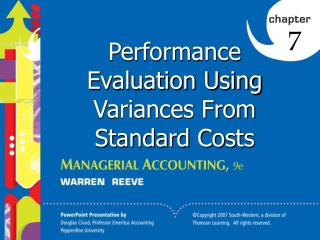DownloadDownload PresentationPerformance Evaluation Using Variances From Standard Costs

# Performance Evaluation Using Variances From Standard Costs

Télécharger la présentation## Performance Evaluation Using Variances From Standard Costs

- - - - - - - - - - - - - - - - - - - - - - - - - - - E N D - - - - - - - - - - - - - - - - - - - - - - - - - - -
##### Presentation Transcript

1. 7 Performance Evaluation Using Variances From Standard Costs

2. 0 7-1 Standards Standards are performance goals. Manufacturers normally use standard costs for each of the three manufacturing costs: • Direct materials • Direct labor • Factory overhead

3. 0 7-2 Standard Cost for XL Jeans 16

4. 0 7-2 Budget Performance Report 19

5. 0 7-2 Relationship of Variances to the Total Manufacturing Cost Variances 20

6. 0 7-3 Direct Materials Standard square yards per pair of jeans 1.50 sq. yards Actual units produced x 5,000 pairs of jeans Standard square yards of denim budgeted for actual production 7,500 sq. yards Standard price per sq. yd. x \$5.00 Standard direct materials cost at actual production \$37,500 22

7. 0 7-3 Direct Materials Price Variance Actual price per unit \$5.50 per sq. yd. Standard price per unit 5.00 per sq. yd. Price variance (unfavorable) \$0.50 per sq. yd. \$0.50 times the actual quantity of 7,300 sq. yds. =\$3,650 unfavorable 23

8. 0 7-3 Direct Materials Quantity Variance Actual quantity used 7,300 sq. yds. Standard quantity at actual production 7,500 Quantity variance (favorable) (200) sq. yds. (200) square yards times the standard price of \$5.00 = (\$1,000) favorable 24

9. Example Exercise 7-1 0 7-3 Tip Top Corp. produces a product that requires six standard pounds per unit. The standard price is \$4.50 per pound. If 3,000 units required 18,500 pounds, which were purchased at \$4.35 per pound, what is the direct materials (a) price variance, (b) quantity variance, and (c) cost variance? 27

10. Follow My Example 7-1 0 7-3 • Direct materials price variance (favorable) • (\$2,775) [(\$4.35 – \$4.50) x 18,500 pounds] • Direct materials quantity variance (unfavorable) • \$2,250 [(18,500 pounds – 18,000 pounds) x • \$4.50] • Direct materials cost variance (favorable) • (\$525) [(\$2,775) + \$2,250] or[(\$4.35 x 18,500 pounds) – (\$4.50 x 18,000 pounds)] = \$80,475 – \$81,000 28 For Practice: PE7-1A, PE7-1B

11. 0 7-3 Direct Labor Variances Standard direct labor hours per pair of XL jeans 0.80 direct labor hour Actual units produced x 5,000 pairs of jeans Standard direct labor hours budgeted for actual production 4,000 direct labor hours Standard rate per DLH x \$9.00 Standard direct labor cost at actual production \$36,000 29

12. 0 7-3 Direct Labor Rate Variance Actual rate \$10.00 Standard rate 9.00 Rate variance—unfavorable \$ 1.00 per hour \$1.00 times the actual time of 3,850 hours =\$3,850 unfavorable 30

13. 0 7-3 Direct Labor Time Variance Actual hours 3,850 DLH Standard hours at actual production 4,000 Time variance—favorable (150) DLH (150) Direct labor hours times the standard rate of \$9.00 =(\$1,350) favorable 31

14. Example Exercise 7-2 0 7-3 Tip Top Corp. produces a product that requires 2.5 standard hours per unit at a standard hourly rate of \$12 per hour. If 3,000 units required 7,420 hours at an hourly rate of \$12.30 per hour, what is the direct labor (a) rate variance, (b) time variance, and (c) cost variance? 34

15. Follow My Example 7-2 0 7-3 • Direct labor rate variance (unfavorable) • \$2,226 [(\$12.30 – \$12.00) x 7,420 hours] • Direct labor time variance (favorable) • (\$960) [7,420 hours – 7,500 hours) x \$12.00] • Direct labor cost variance (favorable) • (\$1,266) [\$2,226 + (\$960)] or [(\$12.30 x 7,420 hours) – (\$12.00 x 7,500 hours)] = \$91,266 – \$90,000 35 For Practice: PE7-2A, PE7-2B

16. 1. Actual variable factory overhead cost greater or less than budgeted variable factory overhead for actual production. 2. Actual production at a level above or below 100% of normal capacity. 0 Variances from standard for factory overhead result from: 7-4 The Factory Overhead Flexible Budget

17. 0 7-4 Variable Factory Overhead Controllable Variance Actual variable factory overhead \$ 10,400 Budgeted variable factory overhead for actual amount produced (4,000 hrs. x \$3.60) 14,400 Controllable variance— favorable \$ (4,000) F 39

18. Example Exercise 7-3 0 7-4 Tip Top Corp. produced 3,000 units of product that required 2.5 standard hours per unit. The standard variable overhead cost per unit is \$2.20 per hour. The actual variable factory overhead was \$16,850. Determine the variable factory overhead controllable variance. 40

19. Follow My Example 7-3 0 7-4 \$350 unfavorable \$16,850 – [\$2.20 x (3,000 units x 2.5 hours)] 41 For Practice: PE7-3A, PE7-3B

20. 0 7-4 Fixed Factory Overhead Volume Variance 100% of normal capacity 5,000 direct labor hours Standard hours at actual production 4,000 Capacity not used 1,000 direct labor hours Standard fixed overhead rate x \$2.40 Volume variance—unfavorable \$ 2,400 U 42

21. Example Exercise 7-4 0 7-4 Tip Top Corp. produced 3,000 units of product that required 2.5 standard hours per unit. The standard fixed overhead cost per unit is \$0.90 per hour at 8,000 hours, which is 100% of normal capacity. Determine the fixed factory overhead volume variance. 44

22. Follow My Example 7-4 0 7-4 \$450 unfavorable \$0.90 x [8,000 hours – (3,000 units x 2.5 hours)] 45 For Practice: PE7-4A, PE7-4B

23. 0 7-4 Reporting Factory Overhead Variances Total actual factory overhead \$22,400 Factory overhead applied (4,000 hours x \$6.00 per hour) 24,000 Total factory overhead cost variance—favorable \$(1,600) F 46